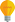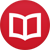# Definite integration without variables

by Jakub MarianTip: See my list of theMost Common Mistakes in English. It will teach you how to avoid mis­takes with com­mas, pre­pos­i­tions, ir­reg­u­lar verbs, and much more.

In the previous article, we discussed how to integrate functions without using variables, but only in the case of indefinite integration. Definite integration is, in fact, just a very simple extension of the indefinite integration theory. All we have to do is to define the operator of limits $[\dots]_a^b$ as follows:

$$\big[F\big]_a^b = F(b)-F(a)\,.$$

The argument of the operator is written inside of square brackets. It would be possible, of course, to devise a separate symbol for the operator and write, say, $I_a^b(F) = F(b)-F(a)$. It is true that this would be more consistent with what we have done so far; however the notation introduced above is customary and will not affect the theory in any way.

Now that we have defined the operator of limits, defining definite integration becomes easy. According to our definition, $∫f$ is the set of all functions $F$ such that $F’ = f$. However, all members of $∫f$ are of the form $F+c$ for some fixed $F$ and $c ∈ ℝ$. Since

$$\big[F+c\big]_a^b = F(b)+c-(F(a)+c) = F(b) - F(a)\,,$$

it is natural to write

$$\l[∫f\r]_a^b = F(b)-F(a) \quad \text{ for any F ∈ ∫f}\,,$$

because the image of the set $∫f$ under $[\dots]_a^b$ is the one element set $\{F(b)-F(a)\}$. And that’s it; $[∫f]_a^b$ is the definite integral of $f$ from $a$ to $b$.

Note: Depending on the theory we want to develop, the definition of $[\dots]_a^b$ can be generalized to $[F]_a^b =$ $\lim_{x→b-} F(x) - \lim_{x→a+}F(x)$, but for the sake of simplicity, we will set $[F]_a^b =$ $F(b)-F(a)$ in this article.

The first obvious advantage is purely notational; if we define $∫_a^b$ to be the Riemann or Lebesgue integral, under certain conditions (such as $f$ being continuous) it holds that

$$∫_a^b f = \l[∫f\r]_a^b\ ,$$

i.e. we have a neat notational tool to distinguish between these two types of integration, which may come in handy in computations. However, the important point is that the $[\dots]_a^b$ operator has its own arithmetical rules. Not only is it linear, i.e. $[F+G]_a^b = [F]_a^b + [G]_a^b$, but it also acts nicely on composition of functions:

$$\big[F[g]\big]_a^b = F(g(b))-F(g(a)) = \big[F\big]_{g(a)}^{g(b)}$$

(where $F[g] = F ∘ g$ is a notation we use for composition). The traditional way to write the substitution rule for definite integration is as follows:

$$∫_a^b f(g(x))g’(x)\,dx = ∫_{g(a)}^{g(b)} f(y)\,dy\,.$$

It is not clear at first sight why the limits changed the way they did (or why the function changed the way it did, for that matter). If you remember the substitution rule for indefinite integration from my previous article, which says that

$$∫f[g]g’ = \l(∫f\r)[g]\,,$$

then the traditional formula becomes apparent:

$$\l[∫f[g]g’\r]_a^b = \l[\l(∫f\r)[g]\r]_a^b = \l[∫f\r]_{g(a)}^{g(b)}\ ;$$

we just used the aforementioned formula for $[F[g]]_a^b$. It was a purely algebraic step, and we do not have to remember the final formula in order to use it in a calculation:

$$\l[∫e^{\sin}\cos\r]_0^{π/2} = \l[\l(∫e^{\iota}\r)[\sin]\r]_0^{π/2} = \big[e^{\iota}\big]_{\sin(0)}^{\sin(π/2)} = \big[e^{\iota}\big]_0^1 = e-1$$

The same works also for the second substitution method, which is traditionally denoted

$$∫_a^b f(x)\,dx = ∫_{g⁻¹(a)}^{g⁻¹(b)} f(g(y))g’(y)\,dy$$

In our new notation, this becomes clear using an in-between step (the inner integral was just rewritten using the substitution rule for indefinite integration):

$$\l[∫f\r]_a^b = \l[\l(∫f[g]g’\r)[g⁻¹]\r]_a^b = \l[∫f[g]g’\r]_{g⁻¹(a)}^{g⁻¹(b)}\ .$$

By the way, I have written several educational ebooks. If you get a copy, you can learn new things and support this website at the same time—why don’t you check them out?